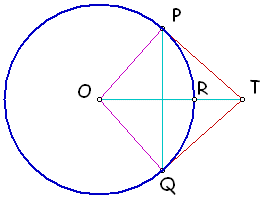#### You may also like### Doodles

Draw a 'doodle' - a closed intersecting curve drawn without taking pencil from paper. What can you prove about the intersections?### Russian Cubes

I want some cubes painted with three blue faces and three red faces. How many different cubes can be painted like that?### Picture Story

Can you see how this picture illustrates the formula for the sum of the first six cube numbers?

# Three Balls

##### Age 14 to 16Challenge LevelA circle has centre O and $\angle POR = \angle QOR.$

Construct tangents at $P$ and $Q$ meeting at $T$.
Draw a circle with diameter $OT$.
Do $P$ and $Q$ lie inside, or on, or outside this circle?
Now imagine a sphere with diameter $OT$.
Do $P$ and $Q$ lie inside, or on, or outside this sphere?